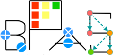Basic Polynomial Algebra Subprograms (BPAS)  v. 1.652
BPASTriangularSet< Field, RecursiveFieldPoly > Member List

This is the complete list of members for BPASTriangularSet< Field, RecursiveFieldPoly >, including all inherited members.

 lower(const Symbol &, BPASTriangularSet< Field, RecursiveFieldPoly > &) const =0 (defined in BPASTriangularSet< Field, RecursiveFieldPoly >) BPASTriangularSet< Field, RecursiveFieldPoly > pure virtual normalForm(const RecursiveFieldPoly &, std::vector< RecursiveFieldPoly > *) const =0 (defined in BPASTriangularSet< Field, RecursiveFieldPoly >) BPASTriangularSet< Field, RecursiveFieldPoly > pure virtual numberOfVariables() const =0 (defined in BPASTriangularSet< Field, RecursiveFieldPoly >) BPASTriangularSet< Field, RecursiveFieldPoly > pure virtual operator=(const BPASTriangularSet< Field, RecursiveFieldPoly > &)=0 (defined in BPASTriangularSet< Field, RecursiveFieldPoly >) BPASTriangularSet< Field, RecursiveFieldPoly > pure virtual operator=(BPASTriangularSet< Field, RecursiveFieldPoly > &&)=0 (defined in BPASTriangularSet< Field, RecursiveFieldPoly >) BPASTriangularSet< Field, RecursiveFieldPoly > pure virtual pseudoDivide(const RecursiveFieldPoly &, std::vector< RecursiveFieldPoly > *, RecursiveFieldPoly *) const =0 (defined in BPASTriangularSet< Field, RecursiveFieldPoly >) BPASTriangularSet< Field, RecursiveFieldPoly > pure virtual select(const Symbol &) const =0 (defined in BPASTriangularSet< Field, RecursiveFieldPoly >) BPASTriangularSet< Field, RecursiveFieldPoly > pure virtual upper(const Symbol &, BPASTriangularSet< Field, RecursiveFieldPoly > &) const =0 (defined in BPASTriangularSet< Field, RecursiveFieldPoly >) BPASTriangularSet< Field, RecursiveFieldPoly > pure virtual variables() const =0 (defined in BPASTriangularSet< Field, RecursiveFieldPoly >) BPASTriangularSet< Field, RecursiveFieldPoly > pure virtual